Next: Enthalpy Up: Classical Thermodynamics Previous: Adiabatic Atmosphere

# Internal Energy

Equation (6.5) can be rearranged to give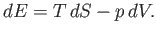(6.82)

This equation shows how the internal energy,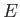, depends on independent variations of the entropy,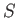, and the volume,. Ifandare considered to be the two independent parameters that specify the system then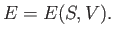(6.83)

It immediately follows that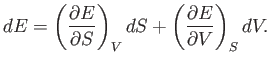(6.84)

Now, Equations (6.82) and (6.84) must be equivalent for all possible values of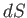and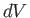. Hence, we deduce that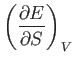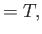(6.85)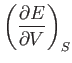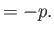(6.86)

We also know that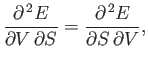(6.87)

or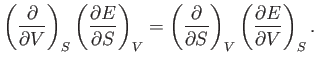(6.88)

In fact, this is a necessary condition for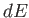in Equation (6.84) to be an exact differential. (See Section 4.5.) It follows from Equations (6.85) and (6.86) that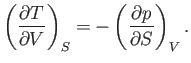(6.89)Next: Enthalpy Up: Classical Thermodynamics Previous: Adiabatic Atmosphere
Richard Fitzpatrick 2016-01-25